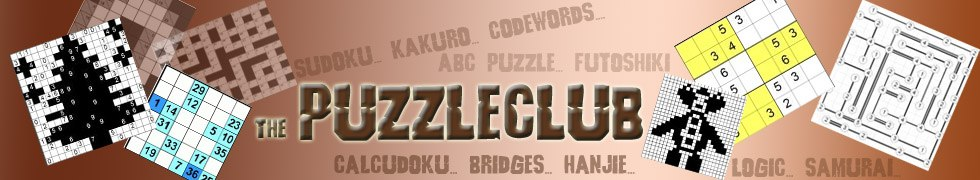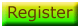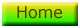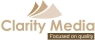# Sample Maths Square

We hope you enjoy this sample maths square puzzle. Just enter the numbers from 1 - 9 into the grid once, following the order of the sums. Full instructions are on the Number Square page: please read these if you are unsure how to play.

If you find it tough to get started, then try to work out which squares are quite restricted in the numbers they can contain, and write in those options in that square: the player will show several numbers in a square at once. Look particularly at high sums or negative sums where the options are option quite constrained, or where there are series of the same type, for instance two division symbols. All answers and answers that form part of the puzzle are always integers - this too can help you reduce the options and work out the answer.

There is only one unique solution to each puzzle and you can work it out with logic; sometimes this is easier than other times but by paying careful attention to the sums and using your knowledge of maths you will get to the answer in the end. For instance, if you have a multiplication sum whose result is odd, then you know that the two numbers that multiply together are odd. So even if you know nothing else about the sum, you've already reduced the options straight down to 1, 3, 5, 7, 9. See if you can come up with similar rules for some other mathematical operations (you can) and soon you'll be well on your way to solving the puzzle.

Here it is - if you get stuck click 'check my solution' and the player will show you if cells do not currently contain the right answer by highlighting those cells. Once you've finished it will tell you if you're right or not. If you get stuck then either reset the puzzle and try again, of if you give up (try not to!) click view solution to see what the correct answer is.

 x - -2 + x - x - 37 / x x + + 12 3 224 36

Check My Solution
View Solution
Reset Puzzle©   |     |